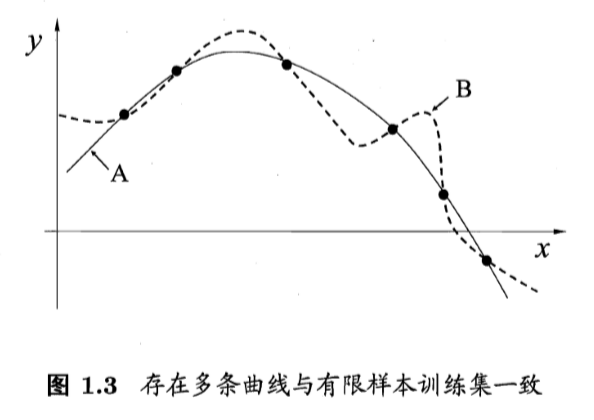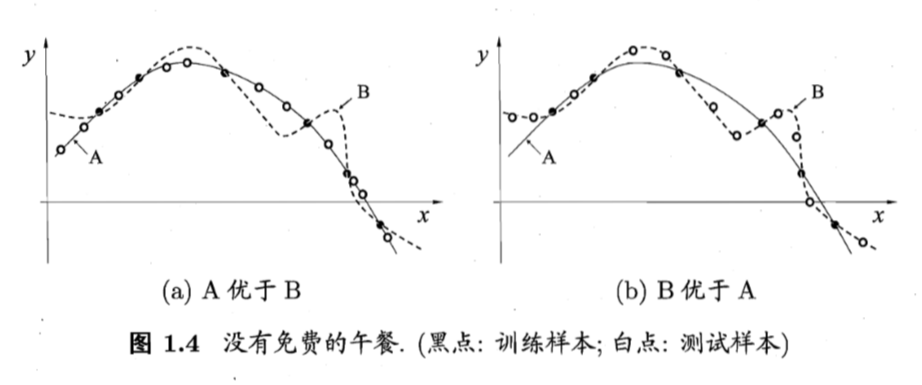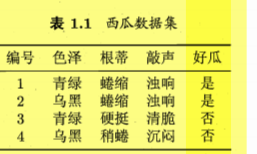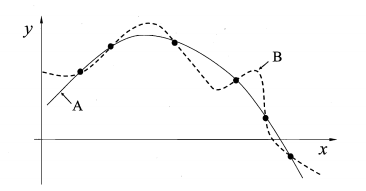• 1.4 归纳偏好   通过学习得到的模型对应了假设空间中的一个假设. 会有多个与训练集一致的假设.如何选择.   机器学习算法在学习过程中对某种类型假设的偏好, 称为 “归纳偏好”(inductive bias), 或简称为"偏好"....
1.4 归纳偏好
通过学习得到的模型对应了假设空间中的一个假设. 会有多个与训练集一致的假设.如何选择.
机器学习算法在学习过程中对某种类型假设的偏好, 称为 “归纳偏好”(inductive bias), 或简称为"偏好". e.g.,若算法喜欢 “特殊” 的模型, 则它会选择 $"好瓜"\leftrightarrow(色泽=*)\bigwedge(根蒂=蜷缩)\bigwedge(敲声=浊响)$;而若算法喜欢 “一般” 的模型. 并且由于某种原因更 “相信” 根蒂, 则它会选择 $"好瓜"\leftrightarrow(色泽=*)\bigwedge(根蒂=蜷缩)\bigwedge(敲声=*)$.
任何一个有效的机器学习算法必有其归纳偏好, 否则它将被假设空间中看似在训练集上 “等效” 的假设所迷惑而无法产生确定的学习结果.
归纳偏好的作用在下面这个学习图示中可能更直观.这里的每个训练样本是图中的一个点$(x,y)$, 要学得一个与训练集一致的模型, 相当于找到一条穿过所有训练样本点的曲线. 对有限个样本点组成的训练集, 存在很多条曲线与其一致. 我们的学习算法必须有某种偏好, 才能产出它认为 “正确” 的模型.归纳偏好可看作学习算法自身在一个可能很庞大的假设空间中对假设进行选择的启发式或 “价值观”. “奥卡姆剃刀”(Occam’s razor)是一种常用的、自然科学研究中最基本的原则, 即 “若有多个假设与观察一致, 则选择最简单的那个”.
对于一个学习算法$\frak L_a$, 若它在某些问题上比学习算法$\frak L_b$好, 则必然存在另一些问题, 在那里$\frak L_b$比$\frak L_a$好.证明: 假设样本空间 $X$ 和假设空间 $H$ 都是离散的. 令
$P(h\mid X,\frak L_a)$ :算法$\frak L_a$基于训练数据 $X$ 产生假设 $h$ 的概率;
$f$: 我们希望学习的真实目标函数;
$\frak L_a$ 的 “训练集外误差” ,即 $\frak L_a$ 在训练集之外的所有样本上的误差为
$E_{ote}(\frak L_a\mid X,f)=\sum _h\sum_{x\in \chi-X} P(x)\Bbb I(h(x)\not=f(x))P(h\mid X,\frak L_a)$,
其中 $\Bbb I(\cdot)$ 是指示函数, 若 $\cdot$ 为真则取值1, 否则取值0.
考虑二分类问题, 且真实目标函数可以是任何函数$X \mapsto \{0,1\}$, 函数空间为$\{0,1\}^{\left|X\right|}$. 对所有可能的 $f$ 按均匀分布对误差求和, 有
$\sum _fE_{ote}(\frak L_a\mid X,f)=\sum_f \sum_h \sum_{x\in \chi-X} P(x)\Bbb I(h(x)\not=f(x))P(h\mid X,\frak L_a)$
$=\sum_{x\in \chi-X}P(x) \sum_hP(h\mid X,\frak L_a) \sum_f \Bbb I(h(x)\not=f(x))$
$=\sum_{x\in \chi-X}P(x) \sum_hP(h\mid X,\frak L_a) \frac122^{\left|\chi\right|}$
$=\frac122^{\left|\chi\right|}\sum_{x\in \chi-X}P(x) \sum_hP(h\mid X,\frak L_a)$
$=2^{\left|\chi\right|-1}\sum_{x\in \chi-X}P(x)\cdot1.$
最终结果显示出, 总误差与学习算法无关! 对于任意两个学习算法 $\frak L_a$ 和 $\frak L_b$, 我们都有
$\sum _fE_{ote}(\frak L_a\mid X,f)=\sum _fE_{ote}(\frak L_b\mid X,f)$ ,
也就是说, 所有学习算法的期望性能都相同. 这就是 “没有免费的午餐” 定理(No Free Lunch Theorem, 简称NFL定理).
然而, NFL定理有一个重要前提: 所有 “问题” 出现的机会相同、或所有问题同等重要. 但实际情形并不是这样. 很多时候, 我们只关注自己正在试图解决的问题(例如某个具体应用任务), 希望为它找到一个解决方案, 至于这个解决方案在别的问题、甚至在相似的问题上是否为好方案, 我们并不关心.
事实上, NFL定理的论述过程中假设了 $f$ 的均匀分布, 而实际情形并非如此.
e.g.,${假设1: 好瓜\leftrightarrow(色泽=*)\bigwedge(根蒂=蜷缩)\bigwedge(敲声=浊响)}$
和
${假设2: 好瓜\leftrightarrow(色泽=*)\bigwedge(根蒂=硬挺)\bigwedge(敲声=清脆)}$
从NFL定理可知, 这两个假设同样好. 然而, $"(根蒂=蜷缩);(敲声=浊响)"$的好瓜很常见, 而$"(根蒂=硬挺);(敲声=清脆)"$的好瓜罕见, 甚至不存在.
所以, NFL定理是让我们清楚地认识到, 脱离具体问题, 空泛地谈论 “什么学习算法更好” 毫无意义. 要谈论算法的相对优劣, 必须要针对具体的学习问题.


展开全文• 1.为什么会产生归纳偏好？ 假设现在有三个与训练集一致的假设，但是与它们对应的模型在面临新的一个样本时，会产生不同的输出。这时明显是不合适的。这时我们需要对其得到的假设进行筛选，因此就产生了归纳偏好.2....
1.为什么会产生归纳偏好？   假设现在有三个与训练集一致的假设，但是与它们对应的模型在面临新的一个样本时，会产生不同的输出。这时明显是不合适的。这时我们需要对其得到的假设进行筛选，因此就产生了归纳偏好.2.深入理解：  实质上，其归纳偏好知识一种判断当一个训练集面对多种假设时的一种思想.上表展示的是一个西瓜的数据集，首先要明确，这是一个二分类问题，我们的目的就是判断一个新的拿来的瓜究竟是不是好瓜，我们将判断好瓜的标准暂且就由三种属性进行判定（色泽，根蒂，敲声）.   现在假设我们训练完成后，有多种对应好瓜的假设。第一种标准(尽可能特殊)：   好瓜<->(色泽=青绿）^(根蒂=蜷缩)^(敲声=浊响)第二种标准（尽可能一般）:   好瓜<->(色泽=*)^(根蒂=蜷缩)^(敲声=*)机器学习对于上面的标准选择的思想就使用了归纳偏好，具体是偏好不同的属性比如在上述的两种标准中，独自偏爱色泽为青绿的这一属性.这就是归纳偏好.另外需要补充的是在实际的应用中，对于不同的属性的偏好选择是应该是基于信赖可视为某种领域知识而产生的归纳偏好，而不是你想当然就视哪一个属性的属性值为你的归纳偏好.3.更加直观的描述（周志华《机器学习》）   假设在下图中，每个训练样本是图中的一个点（x,y）,要学得一个与训练一致的模型，相当于找到一条穿过所有的点的一条曲线,注意，下图中穿过这些样本点的曲线可以有很多种，这就相当于我们的上面所述训练的集合对应的不同的假设，而我们的归纳偏好，就是基于一种偏好选择一种适当的假设。比如：如果我们以最终的曲线要尽量的简洁为一个偏好，那么直观上我们肯定选择下面的曲线A，而不选择曲线B.展开全文• 机器学习（1）归纳偏好和NFL 这是萌新小白第一次写博客，也是刚刚开始机器学习的系统学习。第一次的博客主要介绍的是归纳偏好和NFL定理。 归纳偏好 任何一个有效的机器学习算法都必然有其归纳偏好，否则其就会...
机器学习（1）归纳偏好和NFL
这是萌新小白第一次写博客，也是刚刚开始机器学习的系统学习。第一次的博客主要介绍的是归纳偏好和NFL定理。


归纳偏好

任何一个有效的机器学习算法都必然有其归纳偏好，否则其就会因为假设空间中在训练集上假设的“等效”而感到困惑无法产生确定的学习结果。譬如面对一个有很多西瓜的验证集，对于同样要素（样本特征）的西瓜算法会有时判定为“好瓜”，有时判定为“坏瓜”。这样子的学习结果显然是没有实际作用的。
譬如，训练集中的数据点为

{(0, 0), (1, 1), (-1, 1)}

那么在没有归纳偏好的前提下学习结果可以为

y = 1 - cos(π / 2 * x)

或者为

y = x ^ 2

等等，有无数的曲线可以拟合，也就是作为机器学习第得到的结论，并且这些结论在误差期望上都是相同的（下面的NFL即为证明）。在这种情况下，只有规定了归纳偏好为“平滑”或者其他，才能够得到更为精确的机器学习结果。
归纳偏好可以视为学习算法在自身一个很庞大的假设空间中对假设进行选择的启发式或“价值观”。“奥卡姆剃刀”的简单有效原理便是一种原则。事实上，归纳偏好对应了学习算法本身做出的关于“什么样的模型更好”的假设。具体问题中，归纳偏好是否和问题本身相匹配，大多数时候直接决定了算法能否取得好的性能。

NFL定理

NFL定理证明的是由于对所有可能函数的相互补偿，最优化算法的性能是等价的。该定理暗指，没有其它任何算法能够比搜索空间的线性列举或者纯随机搜索算法更优。该定理只是定义在有限的搜索空间，对无限搜索空间结论是否成立尚不清楚。
NFL定理的结论为：

1）对所有可能的的目标函数求平均，得到的所有学习算法的“非训练集误差”的期望值相同；
2）对任意固定的训练集，对所有的目标函数求平均，得到的所有学习算法的“非训练集误差”的期望值也相同；
3）对所有的先验知识求平均，得到的所有学习算法的“非训练集误差”的期望值也相同；
4）对任意固定的训练集，对所有的先验知识求平均，得到的所有学习算法的的“非训练集误差”的期望值也相同。

NFL定理表明没有一个学习算法可以在任何领域总是产生最准确的学习器。不管采用何种学习算法，至少存在一个目标函数，能够使得随机猜测算法是更好的算法。
首先，假设一个算法为a，而随机胡猜的算法为b，为了简单起见，假设样本空间为 和假设空间为H都是离散的。令P(h|X,a)表示算法a基于训练数据X产生假设h的概率，再令f代表希望的真实目标函数。a的训练集外误差，即a在训练集之外的所有样本上的误差为
$E_{ote}（a|X,f）=\sum_h\sum_{x\isin\chi-X}P(x)Ⅱ(h(x)\neq f(x))P(h|X, a)$
其中，Ⅱ（.）是指示函数，若括号内为真则取值1，否则为0.
考虑二分类问题。且真实目标函数可以使任何函数$\chi\mapsto \{0,1\}$，函数空间为$\{0, 1\}^{|\chi|}$，对所有可能的f按照均匀分布对误差求和，有
\begin{aligned} \sum_f E_{ote}(a, |X,f ) &=\sum_f\sum_h\sum_{x\isin\chi-X}P(x)Ⅱ(h(x)\neq f(x))P(h|X, a) \\ &=\sum_{x\isin\chi-X} P(x)\sum_hP(h|X, a)\sum_fⅡ(h(x)\neq f(x))\\ &=\sum_{x\isin\chi-X} P(x)\sum_hP(h|X, a)*2^{|\chi|-1}\\ &=2^{|\chi|-1}\sum_{x\isin\chi-X} P(x)\sum_hP(h|X, a)\\ &=2^{|\chi|-1}\sum_{x\isin\chi-X} P(x) \end{aligned}
其中最后一步是因为$\sum_hP(h|X, a) = 1$
上式结果证明了，当分布均匀时，也就是没有实际背景时，无论算法多好在没有实际背景情况下都不优于随机胡猜。
所以，NFL定理对于机器学习最重要的寓意，是让我们清晰地认识到，脱离具体问题，空泛地谈论“什么学习算法更好”是没有实际意义的。谈论算法的相对优劣，必须要针对具体的学习问题。大部分学习算法的自身的归纳偏好与问题相匹配时，对于算法性能往往会起到决定性作用。


展开全文• 归纳偏好（inductive bias）：机器学习算法在学习过程中对某种类型假设的偏好。 例如：算法喜欢尽可能特殊的模型，则会选择“好瓜&lt;-&gt;(色泽=*)^(根蒂=蜷缩)^(敲声=浑浊)”，如果算法尽可能一般的模型...
归纳偏好（inductive bias）：机器学习算法在学习过程中对某种类型假设的偏好。

例如：算法喜欢尽可能特殊的模型，则会选择“好瓜<->(色泽=*)^(根蒂=蜷缩)^(敲声=浑浊)”，如果算法尽可能一般的模型，则会选择“好瓜<->(色泽=*)^(根蒂=蜷缩)^(敲声=*)”。

通俗来讲：即在样本空间中存在多种假设，算法选择某种模型假设的偏好。

奥卡姆剃刀：若有多个假设和观察一致，选择最简单的那个。（但有时奥卡姆剃刀也会有不适用，当无法判断哪个更简单时，需要其他机制来解决这个问题）

概括：从假设空间中剪枝，剩余样本空间，样本空间中有多种符合的类型，对某种类型（特殊的，一般的，，，）假设的偏好，就叫归纳偏好。
展开全文• 归纳偏好： 机器学习算法在学习过程中对某种类型假设的偏好，称为“归纳偏好”或简称为“偏好”。 偏好的原则： 奥卡姆剃刀：若有多个假设与观察一致，则选择最简单的那个。 没有免费午餐定理： 首先约定如下： 样本...机器学习
• 任何一个有效的机器学习算法都要有其归纳偏好，否则他将被假设空间中看似在训练集上等效的假设所迷惑。 “奥卡姆剃刀”是一种常用的、自然科学研究中最基本的原则，他能引导算法确立较好的偏好，他的思想是，若有多...机器学习
• 归纳偏好(inductive bias):机器学习算法在学习过程中对某种类型假设的偏好。奥卡姆剃刀(Occam's razor):若有多个假设与观察一致，则选最简单的那一个。“没有免费的午餐”定理(No Free Lunch Theorem 简称NFL定理)...
• 【机器学习杂货铺】——归纳偏好(暂缺) 。。。。。机器学习
• 每种算法必有其归纳偏好，否则它将被假设空间中看似在训练集上“等效”的假设所迷惑，无法产生确定的学习结果。   例子理解： 编号 色泽 根蒂 敲声 好瓜 1 青绿 ...数据挖掘 机器学习 笔记
• 假设空间是指所有可能的能满足样本输入和输出的假设函数h(x)的集合。...这就涉及到归纳偏好的概念了。 　归纳偏好是一个能挑选最佳假设函数的基准。以韦小宝的7个老婆为例，这7个老婆均满足小宝的要求，因此构成机器学习
• 归纳学习有狭义和广义之分，广义的归纳学习大体相当于从样例中学习，而狭义的归纳学习则要求从训练数据中学得概念，也称为概念学习。 概念学习最基本的是布尔概念学习，即对“是”“不是”这样的可表示为0/1布尔值的...
• 归纳偏好 还是举例子： 三种属性：{白色、黄色、黑色}+{眼睛颜色蓝色、眼睛颜色不一样}+{有铃铛、没铃铛} 判断是不是“猫” 通过机器学习，现在给了一幅“白色”“眼睛蓝色”“有铃铛”的动物，让计算机判断...机器学习 西瓜书
• 1.1 超参数 1.2 训练集、验证集、测试集 1.3 归纳偏好 1.4 经验误差与过拟合 1.5 性能度量（performance measure） 1.6 机器学习发展现状机器学习 大数据
• 归纳偏好可看作学习算法自身在一个可能很庞大的假设空间中对假设进行选择的启发式或者价值观。这种价值观的一个典型叫做“奥卡姆剃刀”：若有多个假设与观察一致，则选择最简单那个。 事实上，归纳偏好对应了学习...
• 很显然，机器学习属于归纳的过程，亦称：归纳学习。 以西瓜举例，西瓜成熟与否和西瓜的色泽、根蒂、敲声这三个属性有关系，色泽的属性值：乌黑、青绿。根蒂的属性值：蜷缩、硬挺。敲声的属性值：浊响、沉闷。由这三...
• 一、假设空间   归纳（induction）与演绎（deduction）是科学推理的两个大基本手段。前者是从特殊到一半的...归纳学习有狭义和广义之分，广义的归纳学习大体相当于从样例中学习，而狭义的归纳学习则要求从训练数据
• 我们在机器学习中衡量两个算法，到底孰优孰劣，该怎么考虑？“没有免费的午餐定理”仿佛给了一个很令人 depressing 的答案：针对某一域的所有问题，所有算法的期望性能是相同的。但注意，这里有两个关键词，某一域的...机器学习
• 归纳偏好 归纳偏好对应了学习算法本身所做出的关于“什么样的模型更好”的假设，在具体的现实问题中，假设是否成立，即算法的归纳偏好是否与问题本身的匹配，大多数直接决定了算法能否取得好的性能。 对同样一...人工智能 深度学习 计算机视觉 python 编程语言
• 归纳是特殊到一般的泛化过程 演绎是从一般到特殊的特化过程 概念学习要求从训练数据学得概念 布尔概念学习：对是、不是这样的可以表示成0/1布尔值的目标概念学习 学习的过程可以看作一个在所有假设组成的空间中进行...机器学习
• 参考西瓜书对归纳偏置（偏好）的一些总结理解 模型对应假设空间中的一...归纳偏好可以看作学习算法自身在一个庞大的假设空间中对假设进行选择的启发式或者“价值观”。即天涯何处无芳草，却为什么偏偏选择你！！！机器学习
• 归纳偏好 线性回归 基本形式 线性回归 最小二乘参数估计 对数几率回归 单位阶跃函数 线性判别分析 多分类任务 神经网络 神经元模型 M-P神经元模型 激活函数 感知机与多层网络 误差逆传播算法 全局最小和局部极小 ...机器学习
• 总结归纳假设空间归纳从特殊到一般的“泛化”过程， 即从具体的事实归结出一般性规律...版本空间归纳偏好需要对假设空间进行搜索学习操作的时候，建立一定的偏好（特征选取偏好），这样的学习还有意义。 即， 建立学习机器学习
• 归纳偏置/归纳偏差/inductive bias “归纳偏差”中的“偏差”容易让人想到数据估计中估计值与真实值的差别error，会让人认为“归纳偏差”是某种需要被规避...而“bias”“偏置”是指我们对模型的偏好。因此归纳偏置可
• 机器学习问题假定问题所有的可能解组成一个假设...这些搜索过程的偏好称为归纳偏置。归纳偏置是机器学习的预先假定，是各种算法的本质区别。 这些偏好是否合理成为影响搜索效果的关键因素。所谓合理就是就是偏好...
• ## Git 命令归纳总结

千次阅读 2016-10-13 13:16:03
对于绝大多数工作而言，你可以改变 Git 的默认方式，或者根据你的偏好来设置。 这些设置涵盖了所有的事，从告诉 Git 你的名字，到指定偏好的终端颜色，以及你使用的编辑器。 此命令会从几个特定的配git
• 在上一篇推送中我们总结了机器学习第一课：一些最最基本的概念，比如... 2 归纳偏好归纳偏好（inductive bias），机器学习算法在学习过程中对某种类型假设的偏好。任何一个有效的机器学习算法必有其归纳偏好，否则它机器学习 最小二乘法
• 归纳偏好归纳偏好（inductive bias），机器学习算法在学习过程中对某种类型假设的偏好。任何一个有效的机器学习算法必有其归纳偏好，否则它将被假设空间中看似在训练集上等效的假设所迷惑，而无法生成确定的学习结果...
• 其次，归纳云存储的QoS需求为时间、代价与质量3大类，并据此修改PSO算法的适应度函数用权重因子调节QoS偏好。实验发现，在不同优先级任务分布不均的情况下，分布广的任务的偏好会掩盖其他任务的偏好，因而不适宜用...云存储 粒子群算法 任务调度
• 浅议基于CRM信息技术的渠道偏好度模型的管理应用 4. 渠道模型分析结果与验证 1电话客服中心渠道的偏好度分析在电话客服...通过电话渠道偏好客户分析归纳出影响偏好电话客服渠道最明显的前10个参数如表2示 其中拨打客
• 文章目录一、归纳偏好概念重要性确立正确的偏好的原则没有免费的午餐定理二、模型选择模型选择遇到的困难模型评估性能度量1、分类2、回归3、聚类 一、归纳偏好 概念 归纳偏好指机器学习算法在学习过程中对某种类型...机器学习...